# SSAT Elementary Level Math : How to find the part from the whole

## Example Questions

### Example Question #31 : How To Find The Part From The Whole

Lauren and Juan have 32 blocks. If 20 of those blocks belong to Juan, how many blocks belong to Lauren?Explanation:

We are given the total number of blocks that Lauren and Juan have together, and how many blocks Juan has individually. To find how many blocks Lauren has individually, we should subtract!

Total blocks - Juan's blocks = Lauren's blocks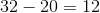### Example Question #32 : How To Find The Part From The Whole

Izzy weighs 81 pounds. If Izzy and Garth weigh 175 pounds together, how much does Garth weigh?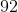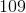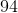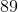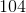Explanation:

We are given the combined weight of Izzy and Garth, and how much Izzy weighs individually. To find how much Garth weighs individually, we should subtract!

Total weight - Izzy's weight = Garth's weight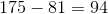### Example Question #33 : How To Find The Part From The Whole

Kim and Stevie have 28 dolls. If 13 of those dolls belong to Kim, how many dolls belong to Stevie?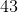Explanation:

We are given the total number of dolls that Kim and Stevie have together and the total number of dolls Kim has individually. To find the number of dolls that Stevie has individually, we need to subtract the number of dolls Kim has from the total number of dolls.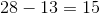### Example Question #31 : How To Find The Part From The Whole

Neda and Sarah havetoy ponies. Ifof these toy ponies belong to Sarah, how many toy ponies belong to Neda?Explanation:

We are given the total number of toy ponies that Neda and Sarah have together, and how many toy ponies Sarah has individually. To find how many toy ponies Neda has individually, we should subtract!

Total toy ponies - Sarah's toy ponies = Neda's toy ponies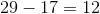### Example Question #32 : How To Find The Part From The Whole

Tony and David have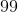toy cars. Ifof these toy cars belong to Tony, how many toy cars belong to David?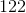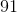Explanation:

We are given the total number of toy cars that Tony and David have together, and how many toy cars Tony has individually. To find how many toy cars David has individually, we should subtract!

Total toy cars - Tony's toy cars = David's toy cars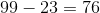### Example Question #4241 : Numbers And Operations

A man won 3 out of 5 games on Monday, 2 out of 7 games on Wednesday, and 3 out of 8 games on Friday. If he only played games on Monday, Wednesday and Friday, what is the ratio of his total wins to losses?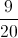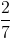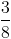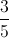Explanation:

The total number of games won by the man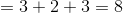.

The total number of games lost by the man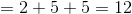.

Therefore, the ratio of wins to losses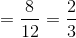.

### Example Question #34 : How To Find The Part From The Whole

Hannah and Oliver havestuffed animals. Ifof those stuffed animals belong to Hannah, how many stuffed animals belong to Oliver?Explanation:

We are given the total number of stuffed animals that Hannah and Oliver have together, and how many stuffed animals that Hannah has individually. To find how many stuffed animals Oliver has individually, we should subtract.

Total stuffed animals – Hannah's stuffed animals = Oliver's stuffed animals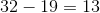### Example Question #35 : How To Find The Part From The Whole

Steven and Mike weigh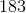pounds together. If Steven weighs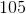pounds, how much does Mike weigh?pounds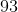pounds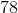pounds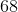pounds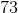poundspounds

Explanation:

We are given the total weight of Steven and Mike, and how much Steven weighs individually. To find how much Mike weighs individually, we should subtract.

Total weight – Steven's weight = Mike's weight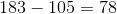### Example Question #31 : How To Find The Part From The Whole

Ruth’s cat weighs 8 pounds. Ruth's friend Lenny also has a cat. If the combined weight of the two cats is 15 pounds, how much does Lenny’s cat weigh?

7.2 pounds

7 pounds

23 pounds

6 pounds

17 pounds

7 pounds

Explanation:

In order to find the weight of Lenny’s cat, subtract the weight of Ruth's cat, 8 pounds, from the combined weight of the two cats, 15 pounds:  15 - 8 = 7 pounds

Lenny's cat weighs 7 pounds.

### Example Question #32 : How To Find The Part From The Whole

Terrance has 14 blue marbles, 5 red marbles, and 19 white marbles. If he lost 8 marbles last week, how many marbles does he have left?

8

28

29

38

30